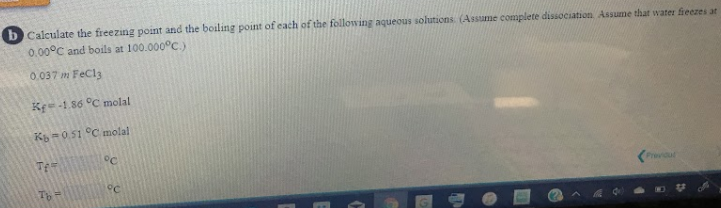# b) Calculate the freezing point and the boiling point of each of the following aqueous solutions. ( Assume complete dissociation. Assume that water freezes at 0.00 degrees C and boils at 100.000 degrees C) 0.037 m FeCl3 Kf = 1.86 degrees C molal Kb = 0.51 degrees C molal Tf = ___ degrees C Tb = ___ degrees C